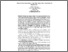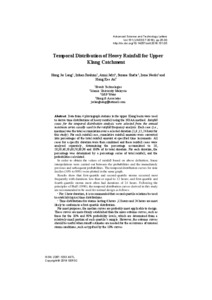# Temporal distribution of heavy rainfall for upper Klang catchment

Hong, Jer Lang and Ibrahim, Izihan and Jafri, Azmi and Shafie, Suzana and Naubi, Irena (2018) Temporal distribution of heavy rainfall for upper Klang catchment. Advanced science and technology letters, 151. pp. 25-30. E-ISSN 2287-1233Preview
PDF - Published Version

## Abstract

Data from 4 pluviograph stations in the upper Klang basin were used to derive time distributions of heavy rainfall using the NOAA method. Rainfall cases for the temporal distribution analysis were selected from the annual maximum series usually used in the rainfall frequency analysis. Each case (i.e., maxima) was the total accumulation over a selected duration (1,6 ,12, 24 hour for this study). For each rainfall case, cumulative rainfall amounts were converted into percentages of the total rainfall amount at specified time increments. All cases for a specific duration were then combined and these rainfall cases were analysed separately, determining the percentage accumulated to 10, 20,30,40,50,60,70,80,90 and 100% of its total duration. For each duration, the percentage was determined by a percentage series of total rainfall, and the probabilities calculated. In order to obtain the values of rainfall based on above definition, linear interpolations were carried out between the probabilities and the immediately previous and subsequent probabilities. The temporal distribution curves for nine deciles (10% to 90%) were plotted in the same graph. Results show that first-quartile and second-quartile storms occurred most frequently with durations less than or equal to 12 hours; and first-quartile and fourth quartile storms most often had durations of 24 hours. Following the principles of Huff (1990), the temporal distribution curves derived in this study are recommended to be used for normal design as follows: • For 1 hour duration, it is recommended that second quartile relations be used to establish typical time distributions. • Time distributions for storms lasting 6 hours ,12 hours and 24 hours are most likely to conform to a first-quartile distribution. For most purposes, the median curves are probably most applicable to design. These curves are more firmly established than the more extreme curves, such as those for the 10% and 90% probability levels, which are determined from a relatively small portion of each quartile’s sample. However, the extreme curves should be useful when runoff estimates are needed for the occurrence of unusual storm conditions, such as typified by the 10% curves.

Item Type: Article (Journal) Rainfall; Temporal Distribution T Technology > TA Engineering (General). Civil engineering (General) > TA170 Environmental engineering. Sustainable engineeringT Technology > TA Engineering (General). Civil engineering (General) > TA197 Engineering meteorologyT Technology > TA Engineering (General). Civil engineering (General) > TA329 Engineering mathematics. Engineering analysisT Technology > TC Hydraulic engineering. Ocean engineering > TC401 River, lake, and water-supply engineering (General) Kulliyyah of Engineering > Department of Civil Engineering Ir. Izihan Ibrahim 05 Aug 2021 10:36 05 Aug 2021 10:36 http://irep.iium.edu.my/id/eprint/65354View Item